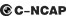## 长安 长安CX208 种颜色可选2014款最低售价：5.59 万元起

3970(mm)1730(mm)1615(mm)##### 配置亮点：
• 胎压监测装置

• ISOFIX儿童座椅接口

• 车身稳定控制(ESC/ESP/DSC等)

• 电动天窗

• 定速巡航

• 后倒车雷达

• 真皮座椅

• GPS导航系统

• 氙气大灯

• 后视镜加热长安 长安CX20 在售车型

排量 车型 厂商指导价 本地最低报价 购车工具
1.4L 1.4L 手动运动版 国IV 5挡手动 5.59万 5.59万

询底价+对比
1.4L 手动天窗导航版 国IV 5挡手动 5.99万 5.99万

询底价+对比
1.4L IMT天窗导航版 国IV 5挡AMT 6.49万 6.49万

询底价+对比
1.4L 手动运动版 国V 5挡手动 5.69万 5.69万

询底价+对比
1.4L 手动天窗导航版 国V 5挡手动 6.09万 6.09万

询底价+对比
1.4L IMT天窗导航版 国V 5挡AMT 6.59万 6.59万

询底价+对比

长安 长安CX20 经销商

查看更多 >>
更多 >>

本地降价排名

### 长安 长安CX20 动力加速

长安CX20 0-100公里加速时间分布在 0.0-秒 属于 超跑级

动力级别 加速时间 车型

长安 长安CX20 视频

长安 长安CX20 新闻资讯

# 长安818汽车节购物攻略 包抢最底价

汽车导购 超过10140次关注

8月18日，长安商城首届汽车节隆重开幕，随着活动上线，多款人气车型及周边产品甚至一上架就被抢购一空。汽车节该怎么玩？小编整理了活动内容以及一些网友们的攻略，...

# 开学座驾你选谁 绅宝D20VS长安CX20

试驾评测 超过8326次关注

又到一年开学季。对于寒窗苦读数十载的学生党来说，终于可以迈入梦寐已久的大学殿堂。在高中被誉为校草的小王，毫无悬念地考上了离家不远的一所重点大学。开学那天...

# 长安星空之旅自由正在路上

试驾评测 超过9394次关注

7日上午9时星空探索团成员从西安市大唐西市博物馆出发，开始了一天的行程。摄像机记下来了CS星空之旅一路上发生的事情，穿梭在这个写满历史的名都，徜徉在经济发展...

# 试驾长安CX20 做工与驾驶感值得肯定

试驾评测 超过6277次关注

长安CX20定位于“跨界多功能性两厢家用轿车”，这样的描述在广告概念泛滥的年代已经很难让人提起兴趣。直到你亲眼看到CX20，你才会理解这一定位的含义，无论是从硬...

# 实拍长安CX20 外观时尚动感 内饰简洁实用

导购 超过5754次关注

作为长安汽车旗下的一款跨界商用小型车，长安CX20自2010年上市以来一直以较为时尚动感的外形以及简洁实用的空间内饰作为卖点。近日，长安CX20在阔别三年之久终于迎...

# C-NCAP碰撞 长安CX20以35.8分获三星

评测 超过4292次关注

C-NCAP碰撞长安CX20以35.8分获三星

# 新款长安CX20正式上市 售5.59万-6.49万

新闻 超过3182次关注

近日我们获得消息，2014款长安CX20正式上市，售价区间为5.59万-6.49万元，共推出4款车型。新车相比现款车型在车身尺寸上有所增加，同时搭载一台1.4L自然吸气发动机。

# 新款长安CX20推三款车型 或成都车展上市

新闻 超过3429次关注

今年上海车展亮相的新款长安CX20，近日有消息曝出将在8月份上市。编辑猜测很有可能在8月底开幕的成都车展中揭开这最终的售价面纱。不过目前可以确认的是，新车将推...

# 长安CX20上市 六款车型售4.99-7.09万

新闻 超过3605次关注

长安汽车旗下首款全民精致拓界车CX20在北京地区宣告上市。此次上市的CX20为1.3LMT和AT，共计六款车型。其中1.3LMT标准型售价4.99万元、1.3LMT舒适型5.39万元、1.3LM...

猜你喜欢

﻿
• 快速找车
• 选择品牌
• 选择品牌
• A  奥迪
• A  阿斯顿·马丁
• A  阿尔法·罗密欧
• B  宝沃
• B  布加迪
• B  巴博斯
• B  保时捷
• B  宾利
• B  奔驰
• B  宝马
• B  本田
• B  别克
• B  标致
• B  比亚迪
• B  宝骏
• B  北汽制造
• B  北汽新能源
• B  北汽幻速
• B  北汽威旺
• B  北京汽车
• B  奔腾
• B  北汽绅宝
• C  长安
• C  长安商用
• C  长城
• C  昌河
• D  大众
• D  道奇
• D  DS
• D  东南
• D  东风风神
• D  东风风行
• D  东风小康
• D  东风风度
• D  东风
• F  福特
• F  丰田
• F  菲亚特
• F  法拉利
• F  福田
• F  福迪
• F  福汽启腾
• G  观致
• G  广汽传祺
• G  广汽吉奥
• G  GMC
• H  红旗
• H  汉腾汽车
• H  哈弗
• H  哈飞
• H  海格
• H  海马
• H  华颂
• H  黄海
• H  华泰
• H  恒天
• J  吉利汽车
• J  捷豹
• J  Jeep
• J  江淮
• J  江铃
• J  金杯
• J  九龙
• J  金旅
• K  凯翼
• K  凯迪拉克
• K  克莱斯勒
• K  科尼塞克
• K  卡威
• K  开瑞
• L  路虎
• L  林肯
• L  劳斯莱斯
• L  兰博基尼
• L  雷克萨斯
• L  铃木
• L  雷诺
• L  理念
• L  力帆
• L  莲花汽车
• L  猎豹
• L  路特斯
• L  陆风
• M  马自达
• M  MG
• M  MINI
• M  玛莎拉蒂
• M  摩根
• M  迈凯轮
• N  纳智捷
• O  欧宝
• O  讴歌
• O  欧朗
• Q  奇瑞
• Q  起亚
• Q  启辰
• R  日产
• R  荣威
• R  瑞麒
• S  三菱
• S  斯威汽车
• S  萨博
• S  smart
• S  斯柯达
• S  斯巴鲁
• S  思铭
• S  双龙
• S  上汽大通
• S  双环
• T  特斯拉
• T  腾势
• W  沃尔沃
• W  五菱汽车
• W  五十铃
• W  威兹曼
• W  威麟
• X  现代
• X  雪佛兰
• X  雪铁龙
• X  西雅特
• Y  一汽
• Y  英菲尼迪
• Y  英致
• Y  依维柯
• Y  野马汽车
• Y  永源
• Z  众泰
• Z  中华
• Z  中兴
• Z  知豆
• 选择车系
• 选择车系
• 车型对比
• 选择品牌
• 选择品牌
• A  奥迪
• A  阿斯顿·马丁
• A  阿尔法·罗密欧
• B  宝沃
• B  布加迪
• B  巴博斯
• B  保时捷
• B  宾利
• B  奔驰
• B  宝马
• B  本田
• B  别克
• B  标致
• B  比亚迪
• B  宝骏
• B  北汽制造
• B  北汽新能源
• B  北汽幻速
• B  北汽威旺
• B  北京汽车
• B  奔腾
• B  北汽绅宝
• C  长安
• C  长安商用
• C  长城
• C  昌河
• D  大众
• D  道奇
• D  DS
• D  东南
• D  东风风神
• D  东风风行
• D  东风小康
• D  东风风度
• D  东风
• F  福特
• F  丰田
• F  菲亚特
• F  法拉利
• F  福田
• F  福迪
• F  福汽启腾
• G  观致
• G  广汽传祺
• G  广汽吉奥
• G  GMC
• H  红旗
• H  汉腾汽车
• H  哈弗
• H  哈飞
• H  海格
• H  海马
• H  华颂
• H  黄海
• H  华泰
• H  恒天
• J  吉利汽车
• J  捷豹
• J  Jeep
• J  江淮
• J  江铃
• J  金杯
• J  九龙
• J  金旅
• K  凯翼
• K  凯迪拉克
• K  克莱斯勒
• K  科尼塞克
• K  卡威
• K  开瑞
• L  路虎
• L  林肯
• L  劳斯莱斯
• L  兰博基尼
• L  雷克萨斯
• L  铃木
• L  雷诺
• L  理念
• L  力帆
• L  莲花汽车
• L  猎豹
• L  路特斯
• L  陆风
• M  马自达
• M  MG
• M  MINI
• M  玛莎拉蒂
• M  摩根
• M  迈凯轮
• N  纳智捷
• O  欧宝
• O  讴歌
• O  欧朗
• Q  奇瑞
• Q  起亚
• Q  启辰
• R  日产
• R  荣威
• R  瑞麒
• S  三菱
• S  斯威汽车
• S  萨博
• S  smart
• S  斯柯达
• S  斯巴鲁
• S  思铭
• S  双龙
• S  上汽大通
• S  双环
• T  特斯拉
• T  腾势
• W  沃尔沃
• W  五菱汽车
• W  五十铃
• W  威兹曼
• W  威麟
• X  现代
• X  雪佛兰
• X  雪铁龙
• X  西雅特
• Y  一汽
• Y  英菲尼迪
• Y  英致
• Y  依维柯
• Y  野马汽车
• Y  永源
• Z  众泰
• Z  中华
• Z  中兴
• Z  知豆
• 选择车系
• 选择车系
• 选择车型
• 选择车型
• 意见反馈